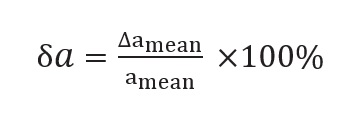## Arithmetic Mean or Average Value:

Let the value obtained in n measurements are a1, a2, a3…., an. Then, the Arithmetic Mean or Average Value of the measurement is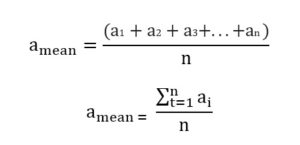Error: The difference between the true value of the quantity and the individual measurement value is called the error of the measurement.
In absence of any other method of knowing true value, we considered arithmetic mean as the true value. Then the errors in the individual measurement values are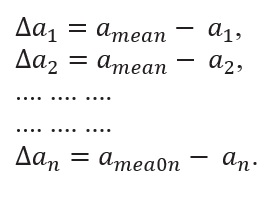The ∆a calculated above may be positive in certain cases and negative in some other cases. The ∆a is also known as the deviation.

## Absolute Error:

The magnitude of the difference between the true value of the quantity and the individual measurement value is called the absolute error of the measurement. That is the absolute value of the deviation is a known as the absolute error.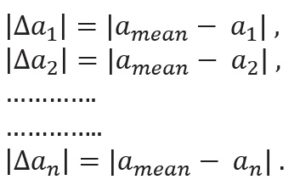The absolute error |∆a| will always be positive.

### Mean Absolute Error:

The arithmetic mean of all the absolute errors is taken as the final or mean absolute error of the value of the physical quantity a. It is represented by Δamean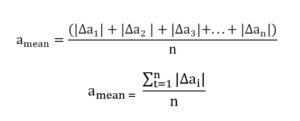Comment: If only a single measurement of the physical quantity a is done by someone else, it is expected to be in the range
amean± ∆amean
i.e. a = amean± ∆amean
that is, the result is expected to be in the range
amean+ ∆amean≤ a ≤ amean– ∆amean

## Relative Error and Percentage Error:

The relative error is the ratio of the mean absolute error ∆amean  to the mean value amean  of the quantity measured.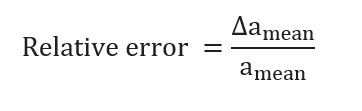When the relative error is expressed in percent, it is called the percentage error a).
Thus, Percentage error